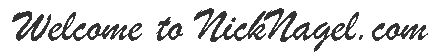﻿ Dr. Nick's Web Tips# SVG Fractals

I put this here as a quick exploration of Fractals and SVG. If you think about it, many classes of fractals are an ideal application of SVG in that SVG defines shapes and operations which readily lend themselves to the application of affine transformations which can be used to create fractal patterns. Throw in ecmascript, and you have a perfect system for the implementation of Iterated Function Systems (IFS's).

### Example: The Sierpinsky Triangle in SVG and Ecmascript

Here's the Sierpinsky Triangle in SVG and ecmascript. Left click the pattern to iterate into the fractal. Right click to reverse. Zoom in and out to see the self-similarity within the figure at different scales.

With respect to transformations, SVG allows you to define groups over which transformations can be applied using matrix operations (as exemplified in the Sierpinsky triangle fractal inlined below). The following code fragment from the example illustrates:

```  <defs>
<polygon
id="iteration_0"
fill="#003399"
points="0,0 2,0 1,1.732" />
<!--
ON EACH ITERATION WE SCALE BY HALF (matrix elements 1 and 4 are
the x and y scaling factors) AND TRANSLATE EACH RESULTANT TRIANGLE
GROUP OUT TO CORNERS...
-->
<g id='iteration_1'>
<use
transform="matrix(0.5 0 0 0.5 0 0)" />
<use
transform="matrix(0.5 0 0 0.5 1  0)" />
<use